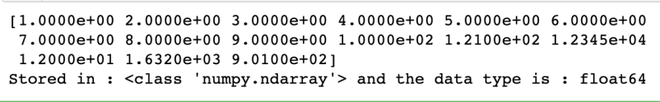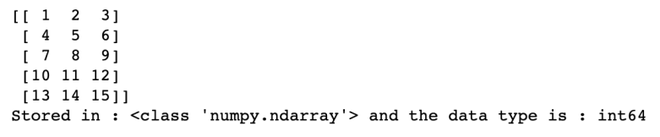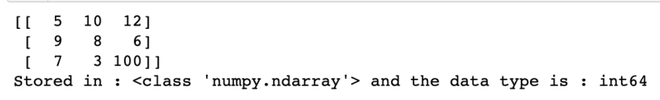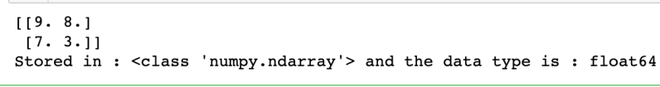Skip to content
Related Articles
How to read a numerical data or file in Python with numpy?
• Last Updated : 03 Mar, 2021

Prerequisites: Numpy

NumPy is a general-purpose array-processing package. It provides a high-performance multidimensional array object and tools for working with these arrays. This article depicts how numeric data can be read from a file using Numpy.

Numerical data can be present in different formats of file :

• The data can be saved in a txt file where each line has a new data point.
• The data can be stored in a CSV(comma separated values) file.
• The data can be also stored in TSV(tab separated values) file.

There are multiple ways of storing data in files and the above ones are some of the most used formats for storing numerical data. To achieve our required functionality numpy’s loadtxt() function will be used.

Syntax: numpy.loadtxt(fname, dtype=’float’, comments=’#’, delimiter=None, converters=None, skiprows=0, usecols=None, unpack=False, ndmin=0)

Parameters:
fname : File, filename, or generator to read. If the filename extension is .gz or .bz2, the file is first decompressed. Note that generators should return byte strings for Python 3k.
dtype : Data-type of the resulting array; default: float. If this is a structured data-type, the resulting array will be 1-dimensional, and each row will be interpreted as an element of the array.
delimiter : The string used to separate values. By default, this is any whitespace.
converters : A dictionary mapping column number to a function that will convert that column to a float. E.g., if column 0 is a date string: converters = {0: datestr2num}. Default: None.
skiprows : Skip the first skiprows lines; default: 0.

Returns: ndarray

### Approach

• Import module
• Load file
• Read numeric data
• Print data retrieved.

Given below are some implementation for various file formats:

Link to download data files used :

Example 1: Reading numerical data from text file

## Python3

 `# Importing libraries that will be used``import` `numpy as np`` ` `# Setting name of the file that the data is to be extarcted from in python``filename ``=` `'gfg_example1.txt'`` ` `# Loading file data into numpy array and storing it in variable called data_collected``data_collected ``=` `np.loadtxt(filename)`` ` `# Printing data stored``print``(data_collected)`` ` ` ` `# Type of data``print``(``    ``f``'Stored in : {type(data_collected)} and data type is : {data_collected.dtype}'``)`

Output :Output of Example 1

Example 2: Reading numerical data from CSV file.

## Python3

 `# Importing libraries that will be used``import` `numpy as np`` ` `# Setting name of the file that the data is to be extarcted from in python``# This is a comma separated values file``filename ``=` `'gfg_example2.csv'`` ` `# Loading file data into numpy array and storing it in variable.``# We use a delimiter that basically tells the code that at every ',' we encounter,``# we need to treat it as a new data point.``# The data type of the variables is set to be int using dtype parameter.``data_collected ``=` `np.loadtxt(filename, delimiter``=``','``, dtype``=``int``)`` ` `# Printing data stored``print``(data_collected)`` ` ` ` `# Type of data``print``(``    ``f``'Stored in : {type(data_collected)} and data type is : {data_collected.dtype}'``)`

Output :Output of Example 2

Example 3: Reading from tsv file

## Python3

 `# Importing libraries that will be used``import` `numpy as np`` ` `# Setting name of the file that the data is to be extarcted from in python``# This``filename ``=` `'gfg_example3.tsv'`` ` `# Loading file data into numpy array and storing it in variable called data_collected``# We use a delimiter that basically tells the code that at every ',' we encounter,``# we need to treat it as a new data point.``data_collected ``=` `np.loadtxt(filename, delimiter``=``'\t'``)`` ` `# Printing data stored``print``(data_collected)`` ` ` ` `# Type of data``print``(``    ``f``'Stored in : {type(data_collected)} and data type is : {data_collected.dtype}'``)`

Output :Output of Example 3

Example 4: Select only particular rows and skip some rows

## Python3

 `# Importing libraries that will be used``import` `numpy as np`` ` `# Setting name of the file that the data is to be extarcted from in python``filename ``=` `'gfg_example4.csv'`` ` `# Loading file data into numpy array and storing it in variable called data_collected``data_collected ``=` `np.loadtxt(``    ``filename, skiprows``=``1``, usecols``=``[``0``, ``1``], delimiter``=``','``)`` ` `# Printing data stored``print``(data_collected)`` ` ` ` `# Type of data``print``(``    ``f``'Stored in : {type(data_collected)} and data type is : {data_collected.dtype}'``)`

Output :Output of Example 4

Attention geek! Strengthen your foundations with the Python Programming Foundation Course and learn the basics.

To begin with, your interview preparations Enhance your Data Structures concepts with the Python DS Course. And to begin with your Machine Learning Journey, join the Machine Learning – Basic Level Course

My Personal Notes arrow_drop_up